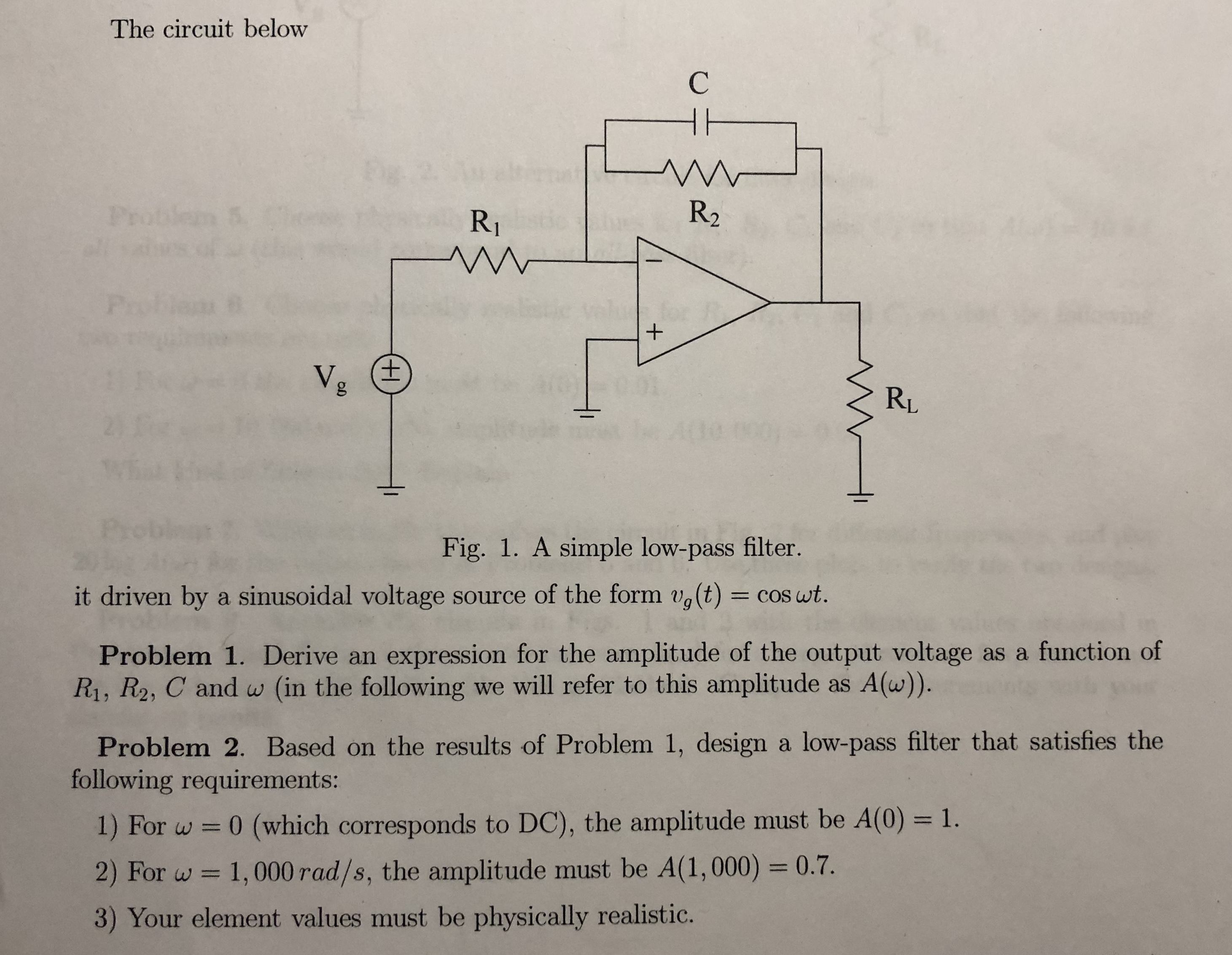# The circuit below R2 RI RL Fig. 1. A simple low-pass filter. it driven by a sinusoidal voltage source of the form ug(t) = cos at Problem 1. Derive an expression for the amplitude of the output voltage as a function of Ri, R2, C and w (in the following we will refer to this amplitude as A(w)). Problem 2. Based on the results of Problem 1, design a low-pass filter that satisfies the following requirements: 1) For w 0 (which corresponds to DC), the amplitude must be A(0) -1. 2) For w 1,000rad/s, the amplitude must be A(1,000) 0.7. 3) Your element values must be physically realistio.

Question

problem 2 question 2help_outlineImage TranscriptioncloseThe circuit below R2 RI RL Fig. 1. A simple low-pass filter. it driven by a sinusoidal voltage source of the form ug(t) = cos at Problem 1. Derive an expression for the amplitude of the output voltage as a function of Ri, R2, C and w (in the following we will refer to this amplitude as A(w)). Problem 2. Based on the results of Problem 1, design a low-pass filter that satisfies the following requirements: 1) For w 0 (which corresponds to DC), the amplitude must be A(0) -1. 2) For w 1,000rad/s, the amplitude must be A(1,000) 0.7. 3) Your element values must be physically realistio. fullscreen

### Want to see the step-by-step answer?